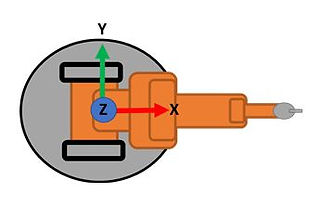top of page# An IntroductionOn this page, I will be continuing on the topic of Rotation Matrices.

In the last page, I introduced some of the basic concpets of Projections and Trigonometric Functions within a Unit Circle to produce Rotation Vectors. The use of these Vectors can be applied to produce a Rotation Matrix.

The aimation below has been used previously to show the capture of frames during the rotation of a robot base.

And using the methods presented int he last page, we can produce the rotation vector of a rotation of axis Z on axis X.

This can be seen more clear in the animation below.But additional to this we can also calculate the vector of a rotation on Z to Y too.

Using the functions within a Unit Circle, we can now explore the rotation of the Y-Axis, the axis perpendicular to the X-axis.

Using the animation below, it becomes visible that the function to calculate the vector for the Y-axis is slightly different. This is because the projection is now in what is called the "Second Quadrant", where the projection is coming from a different direction.Using this simple change, the Rotation Vector for a rotation of Z on Y can be produced as shown in the following figure.And lastly, we can produce the Rotation Vector for a rotation of Z on Z.

Here the vector is extremely simplistic, as there is no projected line and that Z and Z rotated are of the same position and of the same length.

textPutting this all together, we can combine these rotations of Z onto each axis into one Rotation Matrix. This can be used to make or calculate the rotation on all the effected axes at the same time.

Rotation Matrices for rotations of other axis are also easily achievable using similar methods, but the derivation of these matrices is not covered in the scope of this page.On the next page, we will now cover frames further and apply a rotation matrix in software.

The content described so far can be seen in the summary video below.

bottom of page